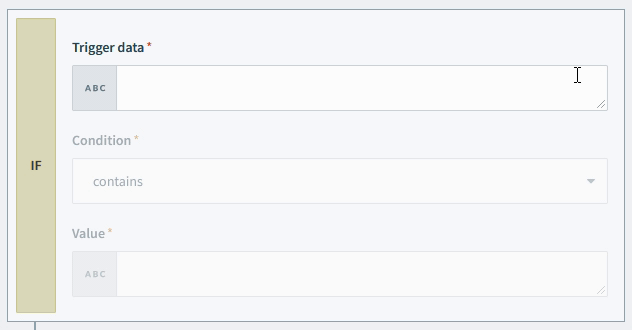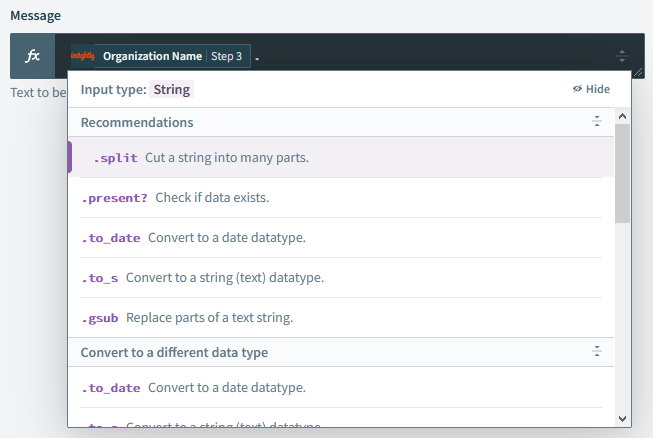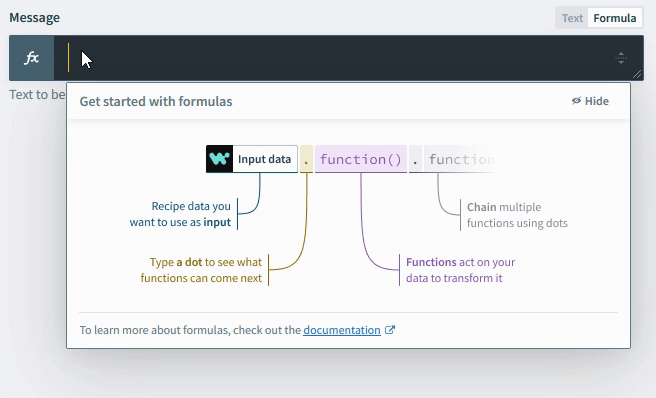# Formulas

Users can use formulas to transform data using AppConnect. Formulas allow users to easily work with and format data. Formulas in AppConnect are whitelisted Ruby methods. Not all Ruby methods are supported, but you can always reach out to us to add additional formulas to the whitelist. Syntax and functionality for these formulas are generally unchanged.

AppConnect allows you to work with the following standard data types:

# Formula mode

To start using formulas, you need to be in formula mode. Formula mode needs to be switched on at the field level, and most input fields support formula mode. To toggle from text mode into formula mode, click on the text/formula mode switch button. This also changes the type icon of the input field into the fx icon.

When the input field is toggled into formula mode, the string type input field changes icon from String type to fx type.# Using the formula editor

In formula mode, the formula editor provides a set of whitelisted formulas available for data transformation/manipulation, and provides additional help on how to use these formulas.

## Filter formulas displayed by data type

The formula editor should always display the type specific formulas for your datapills.

When a string type datapill, the pill Full name, is dropped into an input field in formula mode, the formula editor prompts for a period . to be added behind the pill, as per Ruby syntax. Subsequently, the editor shows the list of formulas applicable to strings.Similarly, when a date type datapill is dropped into the input field in formula mode, the formula editor prompts for a period . to be added behind the pill, subsequently displaying the list of formulas applicable to dates.When there is no datapill within the input field, the general structure of formulas will display, but typing will automatically begin to display other formulas and operators which can be used.## Formula hints and syntax

When a formula is selected to be used, it will be auto-completed in the input field. An explanation of the formula's function, as well as its syntax, will be provided.## Text VS formula mode

In text mode, text and pills can be mapped into the input fields easily, and they will result in a text that looks exactly like what is formed. On the other hand, text needs to be explicitly formatted in formula mode.

Let's use an example of a recipe that sends an email to new leads in Insightly. If a new lead called Madison Diaz is created, we wish to send the following email:

`1Hi Madison,23Thanks for joining our mailing list! We're excited to have you!4`

In text mode, the Message can simply be formed like this:In formula mode, text must have proper string syntax:## Conditionals

You can conditionally execute formulas using Ruby's ternary syntax (popular shortcut for if-else statements). Ternary syntax are of the form:

`1condition ? expression1 : expression22`

### Behaviour

#### condition

A boolean expression that evaluates to true or false.

#### expression1

Returns this expression if condition is true.

#### expression2

Returns this expression if condition is false.

### Conditional formula example

In the following example, we conditionally pass in either the Full name or First name into the Message input field.

Checks if Full name is present. Outputs Full name if present, or First name if not present.

Here is a detailed explanation of what the ternary formula does:

1. Full name.present? will check if the Full name pill has a value . If it has a value, it evaluates to true. If it has no value, it evaluates to false.

2. The second ? in the formula separates the condition to evaluate from the expressions to return. Note, the first ? is part of the .present? formula whilst the second ? is separated with a space character and is part of the ternary syntax.

3. If there is a value in the Full name pill when the job is ran, the value for Full name will mapped to the Message input.

4. If there is no value in the Full name pill when the job is ran, the value for First name will be mapped to the Message input. Of course, if there's also no value in this First name pill, the job will fail at this step if Message is a required input field.

Checks if the input is valid. If true, it performs a specified operation on the input data.

### Syntax

Input and operation

• Input - An input datapill. It can by any datatype.

• Operation - If the input is not null, this formula will be applied to the input data. This formula must be compatible with the input datatype.

### How it works

The Safe Navigation Operator (&.) checks if the input data is valid. It returns a null value if the input data is null or undefined. If the input is valid, it will perform the operation specified by the operation argument. It enables you to write simpler expressions to handle null values.

• Before

The Created Date datapill needs to be converted to a date datatype using the to_date formula. Since the formula will encounter an error if the datapill contains null value, you would have to use a ternary expression to work around this logic.Using ternary conditions

• After

Use the &. operator instead. The safe navigation operator allows you to manage cases where Created Date contains a null value, while writing a simpler formula expression.Using Save nagivation operator